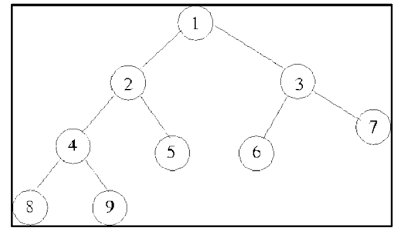# C++ program to Replace a Node with Depth in a Binary Tree

Suppose we have a binary tree and we want to replace the depth of each node with its value. The depth of the node starts from 0 at the root node and increases by 1 for each level we go; for example, we have a binary tree like this;Here we replace,

Node Value Depth
1 0
2 1
3 1
4 2
5 2
6 2
7 2
8 3
9 3

We do a simple preorder tree traversal and assign each node the depth.

Let’s look at some input scenarios −

Suppose we have values of nodes in binary tree as [3 2 7 5 4 6 9 7 2] and the output obtained from this input would be −

Input: [3 2 7 5 4 6 9 7 2]
Result:
Before: 6 5 9 2 4 3 7 7 2
After: 3 2 3 1 2 0 2 1 2


Suppose we have values of nodes in binary tree as [4 3 5 6 10 7 12 8 1] and the output obtained from this input would be −

Input: [4 3 5 6 10 7 12 8 1]
Result:
Before: 7 6 12 3 10 4 8 5 1
After: 3 2 3 1 2 0 2 1 2


## Example

Following is a C++ program to replace a node value in a binary tree with its corresponding depth within the tree −

#include <iostream>
using namespace std;
class Node {
public:
int value;
Node *left, *right;
Node(int value) {
this->value = value;
left = right = NULL;
}
};
void solve(Node *node, int depth) {
if (node == NULL) {
return;
}
node->value = depth;
solve(node->left, depth+1);
solve(node->right, depth+1);
}
void printInorder(Node* root) {
if (root == NULL) {
return;
}
printInorder(root->left);
cout << root->value <<" ";
printInorder(root->right);
}
int main() {
Node* root = new Node(1);
root->left = new Node(2);
root->right = new Node(3);
root->left->left = new Node(4);
root->left->right = new Node(5);
root->left->left->left = new Node(8);
root->left->left->right = new Node(9);
root->right->left = new Node(6);
root->right->right = new Node(7);
cout << "Before: ";
printInorder(root);
solve(root, 0);
cout << "\nAfter: ";
printInorder(root);
return 0;
}


## Output

Before: 8 4 9 2 5 1 6 3 7
After: 3 2 3 1 2 0 2 1 2


## Conclusion

We conclude that using a simple preorder traversal of the tree and replacing the value with depth at each node is sufficient to solve the problem. There is an O(n) time complexity. Here is a article we hope will be helpful to you.